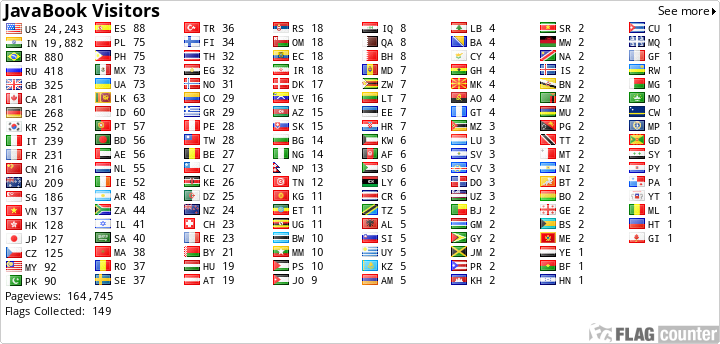### Index Joining techniques

1.nested loop join
2.sort merge join
3.hash join
4.cartesian product
Note : If there is no joining condition, oracle evaluates to cartesian product.

SQL>select empno,ename,deptno,dname from emp,dept where emp.deptno=dept.deptno; --->wrong
SQL>select empno,ename,dname from emp,dept; -->14*4 --> correct
Different types of joining techniques used by developers are
1.equi join
2.non equi join
3.left outer join
4.right outer join
5.full outer join

Nested Loop Join : Optimizer considers nested loop join if the volume of data is very less in the joining tables. Nested loop means, loop within a loop which means there will be outer loop as well as inner loop;
SQL>select e.empno,e.ename,d.deptno,d.dname from emp e,dept d where e.deptno=d.deptno;

Note :  We can also change the behaviour of the optimizer with the help of hints.
example :
ordered
use_NL
use_merge
use_hash
index_ffs

for i in(select * from emp)
loop
(for j in(select * fromm dept where i.deptno=j.deptno)
loop
display result t;
end loop;
end loop;

SQL>select /* +ordered use_NL(d) */ e.empno,e.ename,d.deptno,d.dname from emp e,dept d where e.deptno=d.deptno;
Note : In case of nested loop, recommanded to create index on the table used in the inner loop.

Sorted Merge Join : Optimizer considers sort merge join if the volume of data is moderate.
Optimizer goes for it if there is no equi join(>=,<=,>,<).

Hash Join : Optimizer considers hash join if we are joining a small table with a table where huge volume of data is available.
Hash join works in 2 phases
1.build phase
2.probe phase
In the build phase, oracle creates a hash table in the hash memory by considering the smallest table.
In the probe phase, for every record in the bigger table, oracle probes for a record in the hash table.